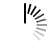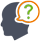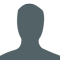Diwali Bonanza | Get 30-Days Free Fun-Filled Learning | Subscribe Now1800-833-6464 Buy Activation Why Tutorix Features Login

#DoubtTushar

In figure below, $∠\ ABC\ =\ 90^o$ and $BD\ ⊥\ AC$. If $AC\ =\ 5.7\ cm$, $BD\ =\ 3.8\ cm$ and $CD\ =\ 5.4\ cm$, find $BC$.Solutions :Akhilesh

Given:

In the given figure $∠ \ ABC\ =\ 90^o$ and $BD\ âŠ¥\ AC$.

$AC\ =\ 5.7\ cm$, $BD\ =\ 3.8\ cm$ and $CD\ =\ 5.4\ cm$.

To do:

We have to find $BC$.

Solution:

In $\vartriangle ABC$ and $\vartriangle BDC$,

$\angle ABC=\angle BDC=90^o$

$\angle C=\angle C$   (common)

Therefore,

$\vartriangle ABC∠¼\vartriangle BDC$  (By AA similarity)

$\frac{AB}{BD} = \frac{BC}{DC}$   (Corresponding parts of similar triangles are proportional)

$\frac{5.7}{3.8} = \frac{BC}{5.4}$

$BC = \frac{5.7\times 5.4}{3.8}$

$BC = \frac{16.2}{2} = 8.1\ cm$

The measure of $BC$ is $8.1\ cm$.

0

﻿